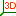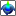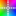## Plot 3D diagram in Pandat

In PandatTM software, you can not only plot typical 2D phase diagrams (Plot diagrams from table in Table options), but also some 3D line graphs, surface graphs and color map graphs.

You can plot 3D phase diagram or color map graphs from any table or HTC result report table. The first click select column is set as x-axis, then hold the “Ctrl” key on the keyboard, the second selected column is set as Y-axis, keep holding the “Ctrl” key, the last selected column is the Z-axis in 3D graphs. Please note that the X, Y, Z axis is determined by the sequence of the selection, not related to the position of the columns in the table.

Figure 1. Select three columns from table data for plotting.

Then you click the icons from toolbar to plot different types of 3D phase diagram.icon for 3D line graph;icon for 3D surface graph; andicon for color map graph. Different types of diagrams are shown in Figure 1.

Figure 2a. 3D line graph of Mg-Al-Zn liquidus lines.

Figure 2b. 3D surface graph of Mg-Al-Zn liquidus surface.

Figure 2c. The color map of Mg-Al-Zn liquidus projection.